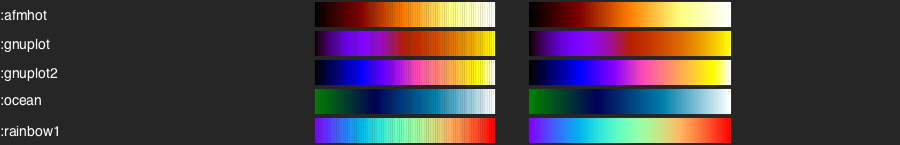# Basics

## ColorScheme objects

When you start using ColorSchemes.jl, it loads a set of pre-defined ColorSchemes, and stores them in a dictionary called colorschemes.

A ColorScheme is a Julia object which contains:

• an array of colors
• a string defining a category
• a string that can contain descriptive notes

To access one of these built-in colorschemes, use its symbol:

julia> ColorSchemes.leonardo

If you're using Juno, for example, the colors in the colorscheme should appear in the Plots window.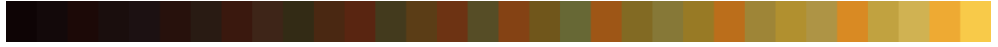Otherwise, you'll see the colors listed as RGB values:

32-element Array{RGB{Float64},1}:
RGB{Float64}(0.0548203,0.016509,0.0193152)
RGB{Float64}(0.0750816,0.0341102,0.0397083)
RGB{Float64}(0.10885,0.0336675,0.0261204)
RGB{Float64}(0.100251,0.0534243,0.0497594)
...
RGB{Float64}(0.620187,0.522792,0.216707)
RGB{Float64}(0.692905,0.56631,0.185515)
RGB{Float64}(0.681411,0.58149,0.270391)
RGB{Float64}(0.85004,0.540122,0.136212)
RGB{Float64}(0.757552,0.633425,0.251451)
RGB{Float64}(0.816472,0.697015,0.322421)
RGB{Float64}(0.933027,0.665164,0.198652)
RGB{Float64}(0.972441,0.790701,0.285136)

You can access the array of colors as:

ColorSchemes.leonardo.colors

By default, the colorschemes aren't imported. But to avoid using the prefixes, you can import the ones that you want:

julia> import ColorSchemes.leonardo
julia> leonardo
32-element Array{RGB{Float64},1}:
RGB{Float64}(0.0548203,0.016509,0.0193152)
RGB{Float64}(0.0750816,0.0341102,0.0397083)
RGB{Float64}(0.10885,0.0336675,0.0261204)
RGB{Float64}(0.100251,0.0534243,0.0497594)
...
RGB{Float64}(0.757552,0.633425,0.251451)
RGB{Float64}(0.816472,0.697015,0.322421)
RGB{Float64}(0.933027,0.665164,0.198652)
RGB{Float64}(0.972441,0.790701,0.285136)

You can reference a single value of a scheme once it's loaded:

leonardo

-> RGB{Float64}(0.10884977211887092,0.033667530751245296,0.026120424375656533)

Or you can 'sample' the scheme at any point between 0 and 1 using get or getindex:

get(leonardo, 0.5)

-> RGB{Float64}(0.42637271063618504,0.28028983973265065,0.11258024276603132)

leonardo[0.5]

-> RGB{Float64}(0.42637271063618504,0.28028983973265065,0.11258024276603132)
Base.getFunction
get(cscheme::ColorScheme, inData :: Array{Number, 2}, rangescale=:clamp)
get(cscheme::ColorScheme, inData :: Array{Number, 2}, rangescale=(minVal, maxVal))

Return an RGB array of colors generated by applying the colorscheme to the 2D input data.

If rangescale is :clamp the colorscheme is applied to values between 0.0-1.0, and values outside this range get clamped to the ends of the colorscheme.

Else, if rangescale is :extrema, the colorscheme is applied to the range minimum(indata)..maximum(indata).

Examples

img = get(colorschemes[:leonardo], rand(10,10)) # displays in Juno Plots window, but
save("testoutput.png", img)      # you'll need FileIO or similar to do this

img2 = get(colorschemes[:leonardo], 10.0 * rand(10, 10), :extrema)
img3 = get(colorschemes[:leonardo], 10.0 * rand(10, 10), (1.0, 9.0))

# Also works with PerceptualColourMaps
using PerceptualColourMaps # warning, installs PyPlot, PyCall, LaTeXStrings
img4 = get(PerceptualColourMaps.cmap("R1"), rand(10,10))
get(cs::ColorScheme, g::Color{T,1} where T<:Union{Bool, AbstractFloat, FixedPoint})

Return the color in cs that corresponds to the gray value g.

## The colorschemes dictionary

The ColorSchemes module automatically provides a number of predefined schemes. All the colorschemes are stored in an exported dictionary, called colorschemes.

colorschemes[:summer] |> show
ColorScheme(
ColorTypes.RGB{Float64}[
RGB{Float64}(0.0,0.5,0.4),
RGB{Float64}(0.01,0.505,0.4),
RGB{Float64}(0.02,0.51,0.4),
RGB{Float64}(0.03,0.515,0.4),
...
RGB{Float64}(1.0,1.0,0.4)],
"matplotlib",
"sampled color schemes, sequential linearly-increasing shades of green-yellow")

## Pre-defined schemes

The left swatch draws the colors in each scheme; the right swatch samples the scheme from 0 to 1 at intervals of 0.01.

## ✦ cmocean

From "Beautiful colormaps for oceanography": cmocean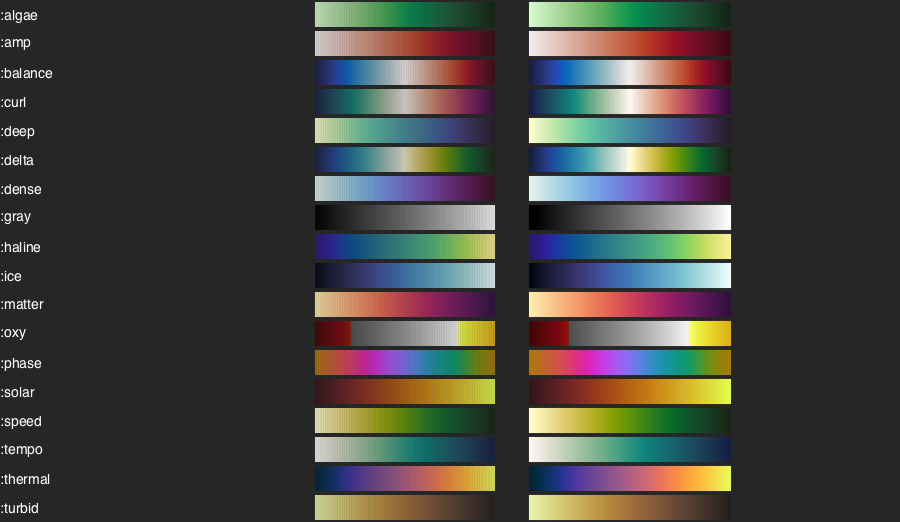## ✦ scientific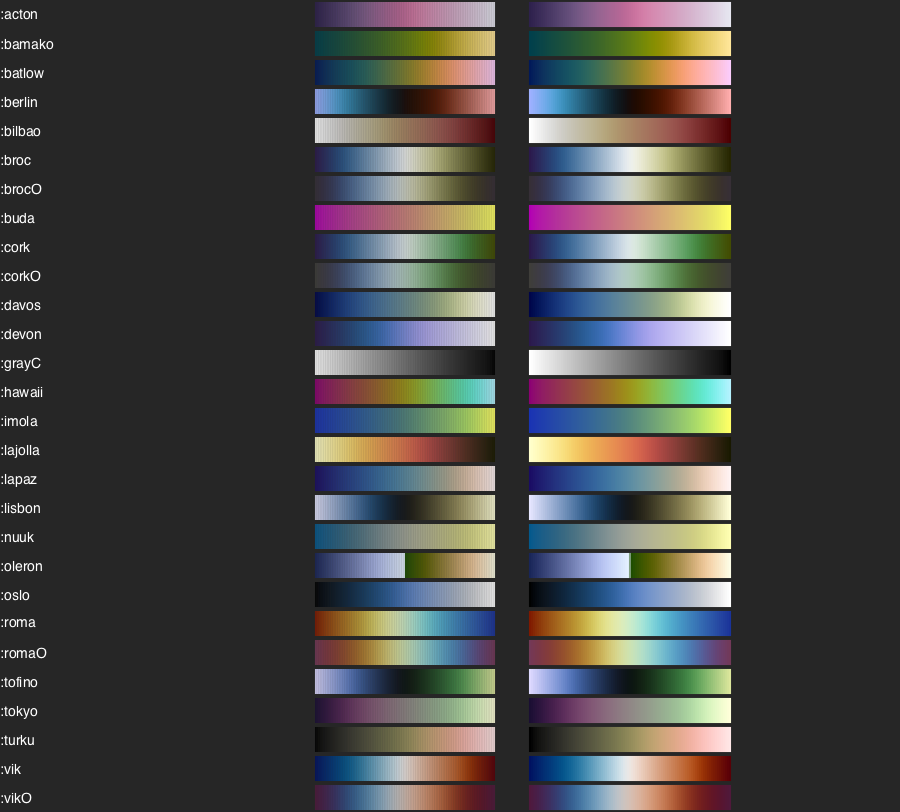## ✦ matplotlib

From matplot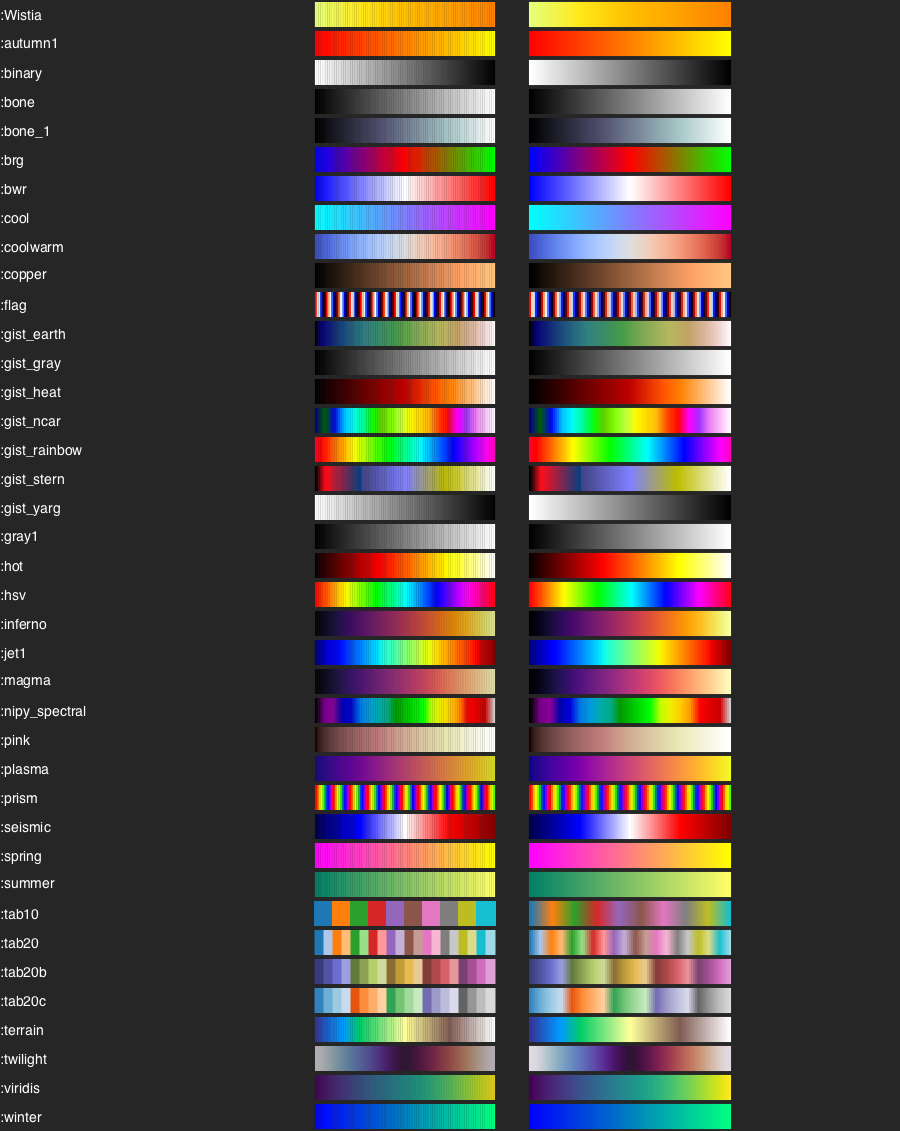## ✦ colorbrewer

From ColorBrewer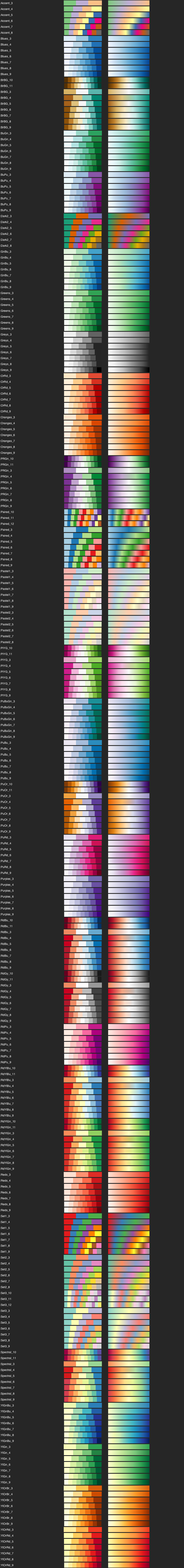## ✦ gnuplot

From GNUPlot